# Inter Maths solutions for Differentiation exercise 9(a)

Intermediate mathematics 1B Differentiation exercise 9(a) all problems with solutions are given.

First you study textbook very well. Observe the example problems and solutions given in the textbook.

Observe the given below problems and solutions and try them in your own method.

You can also see

Inter Maths 1A textbook solutions

Inter Maths 1B textbook solutions

Inter Maths IIA textbook solutions

Inter Maths IIB textbook solutions

Differentiation

Exercise 9(a)

Exercise 9(b)

Exercise 9(c)

Exercise 9(d)

M

## Exercise 9(a) Differentiation solutions Inter maths 1B

Chapter – 9 Differentiation

Exercise 9(a)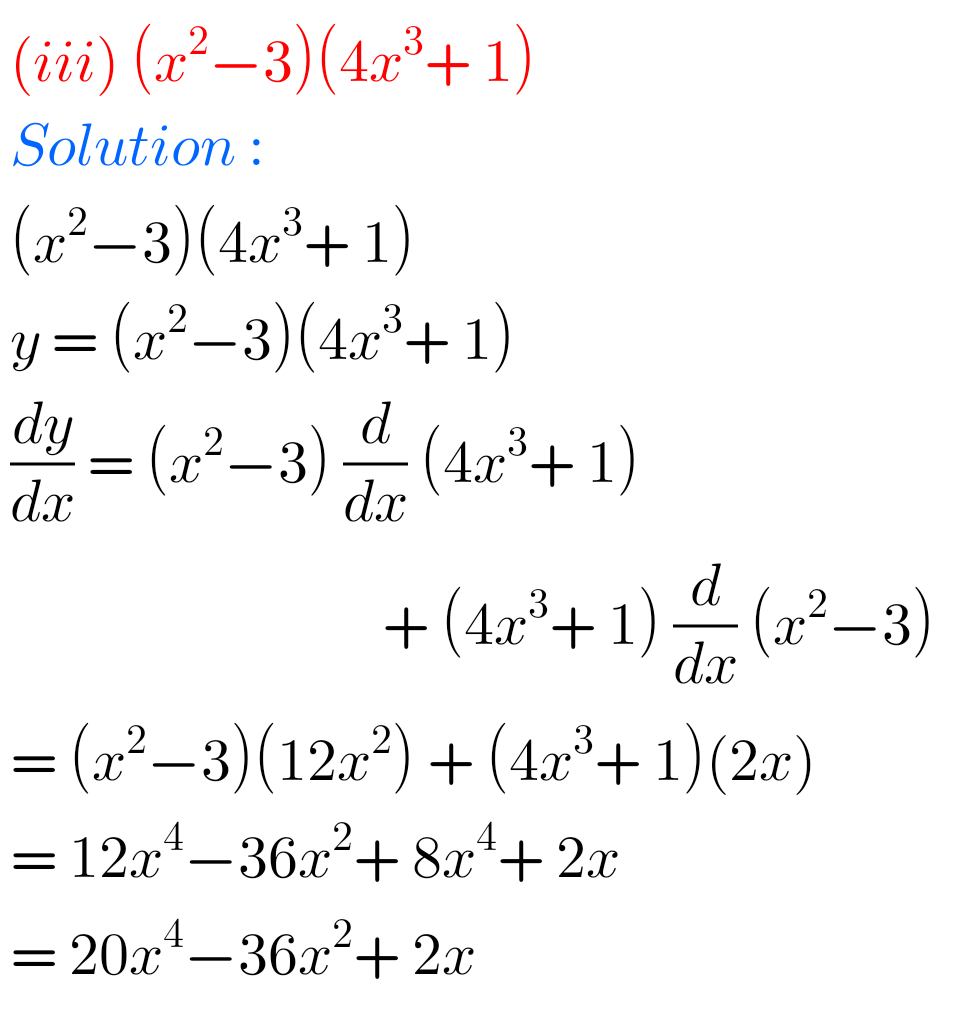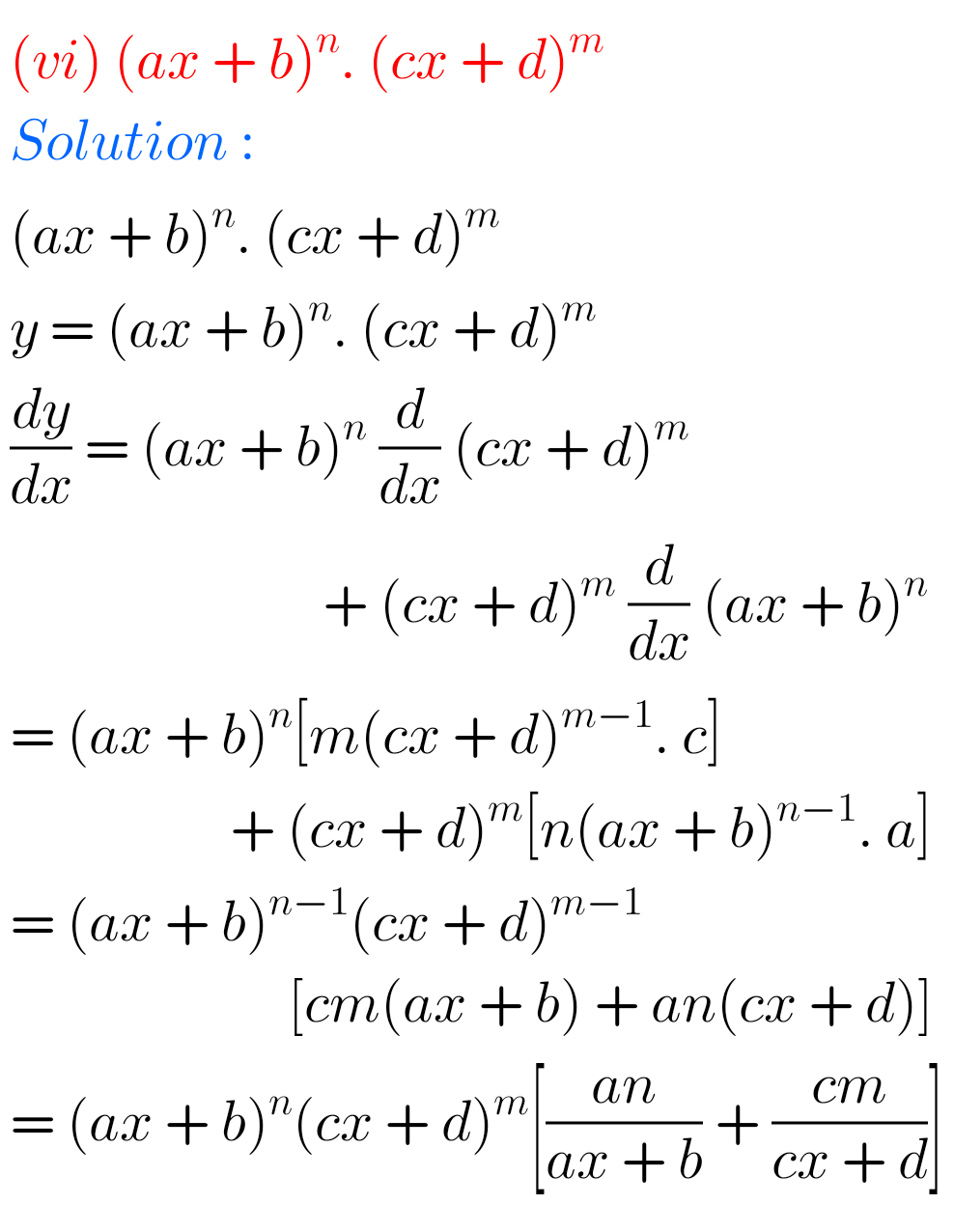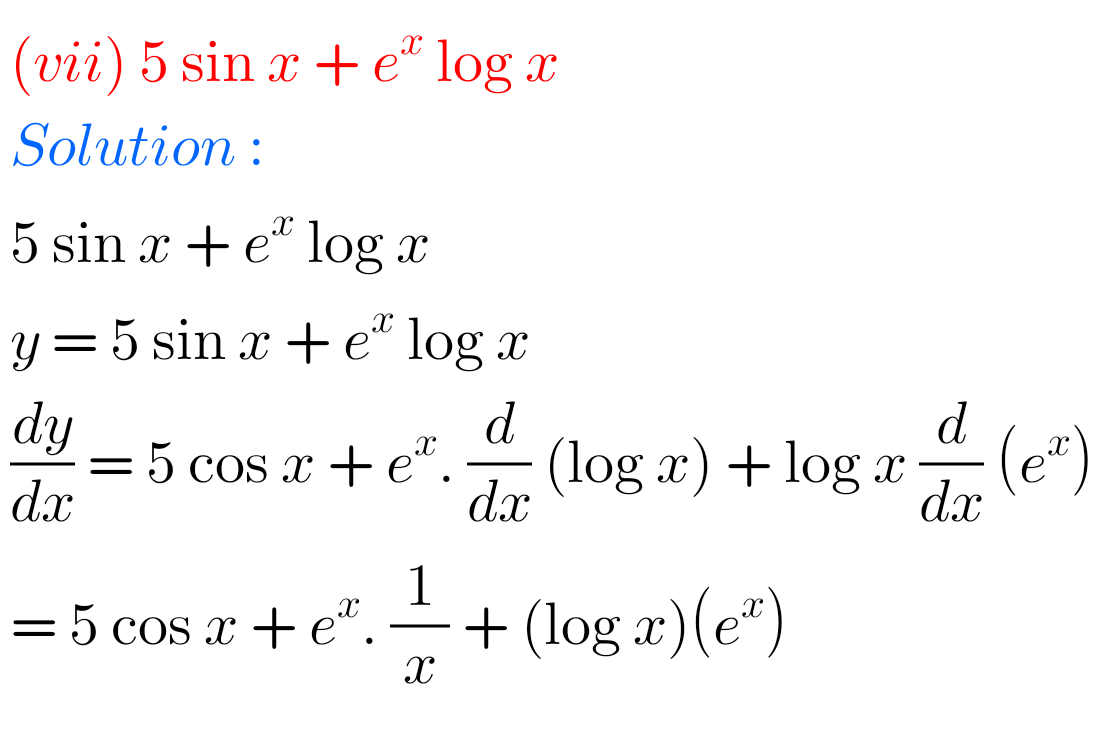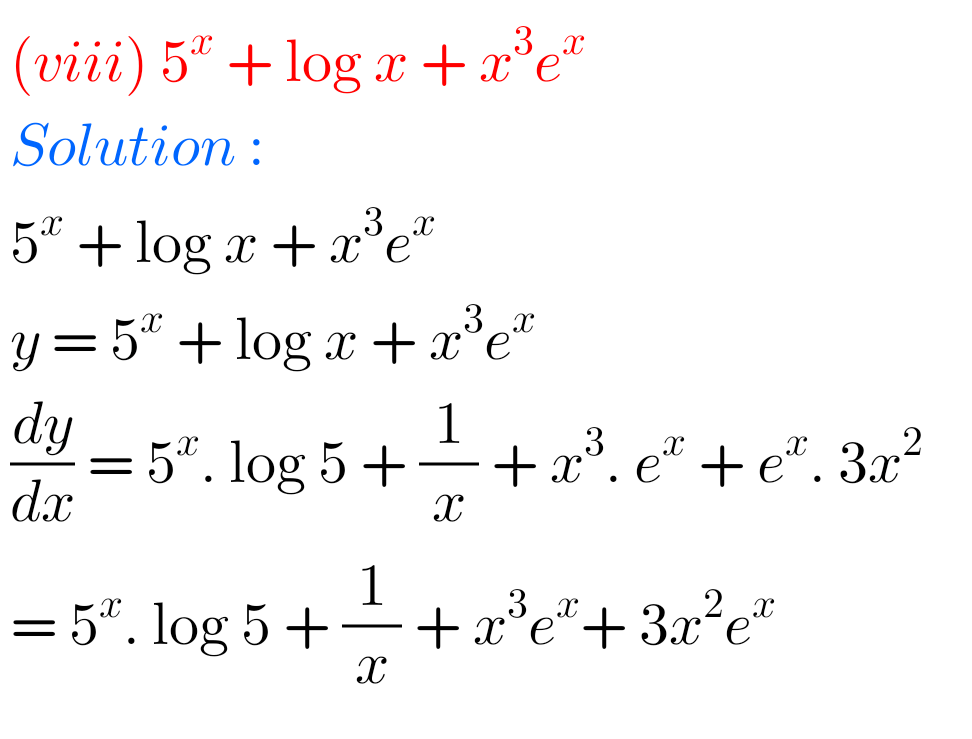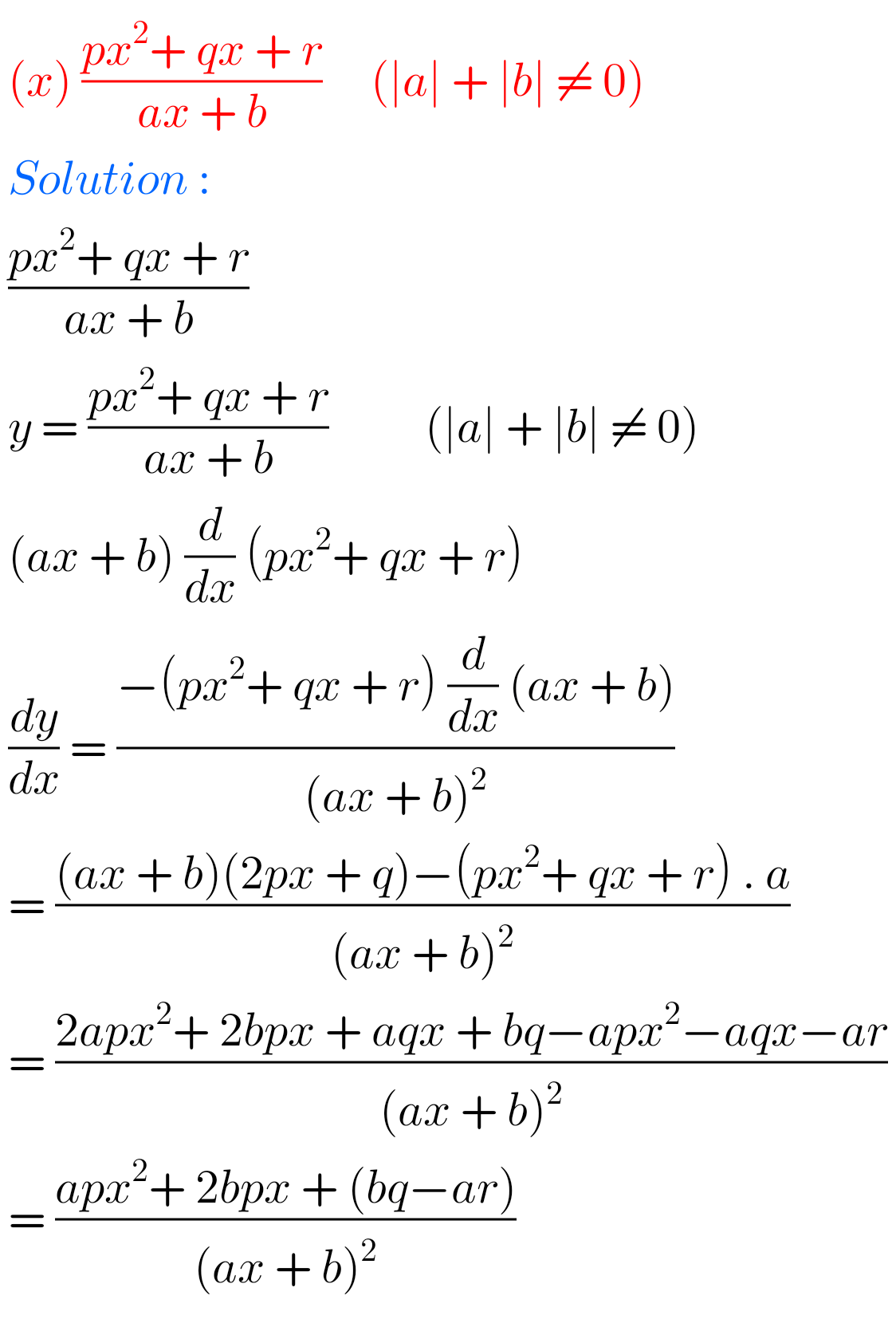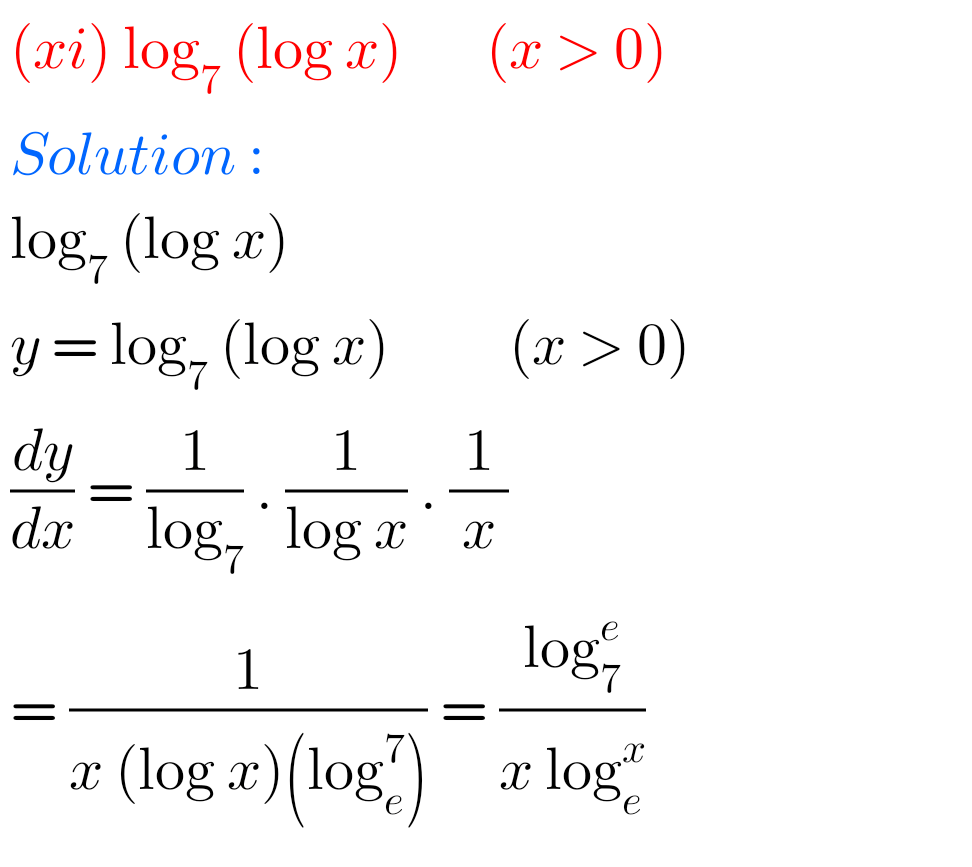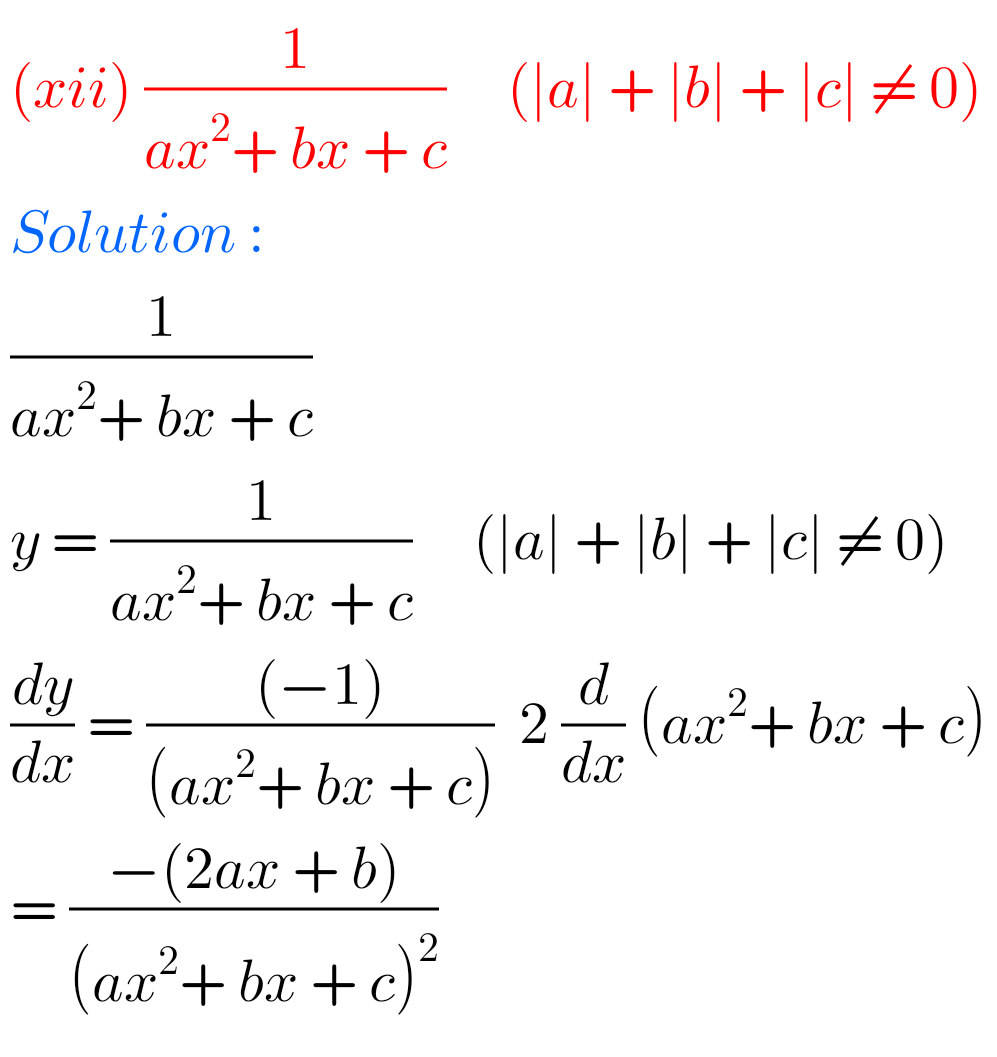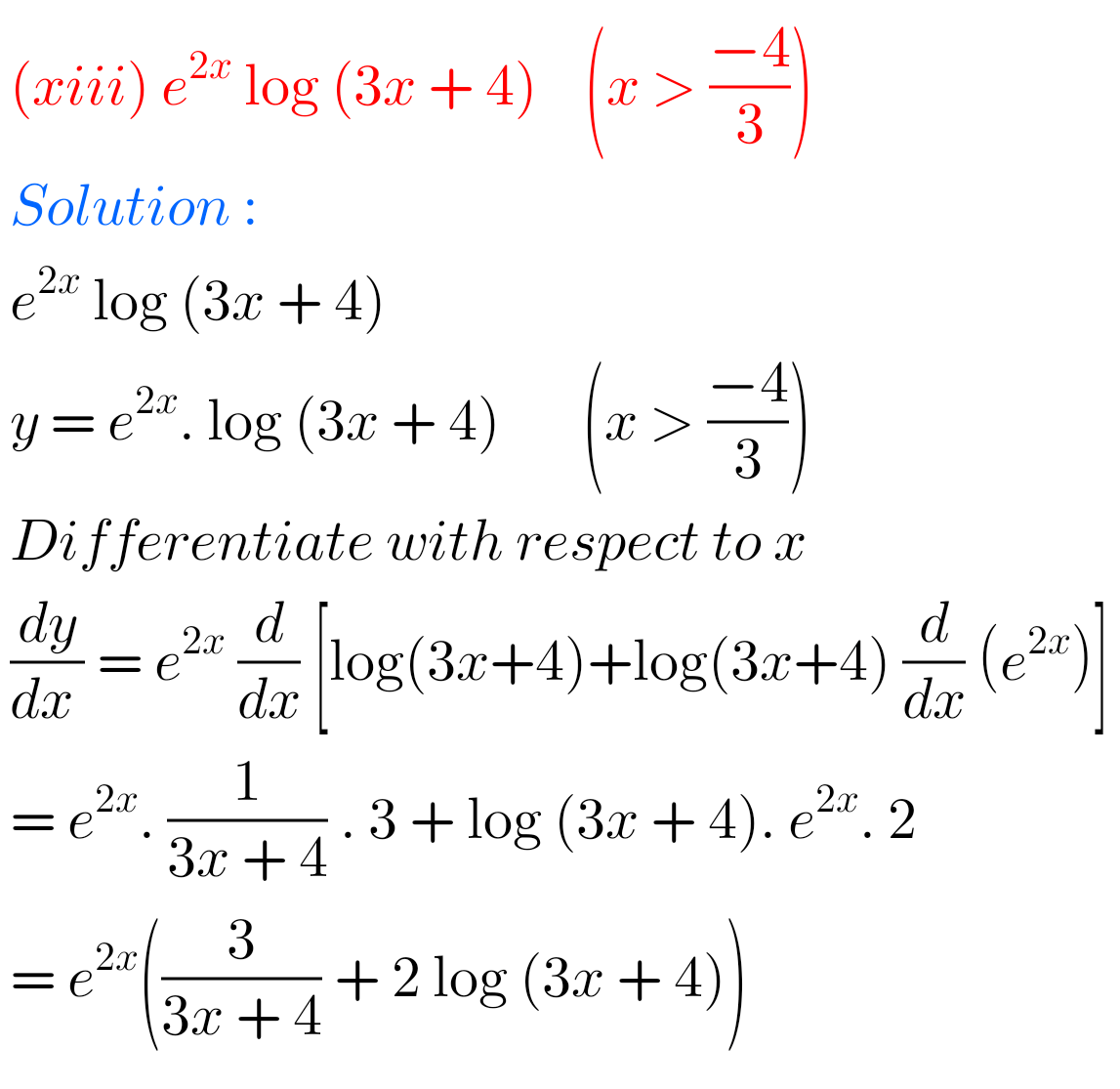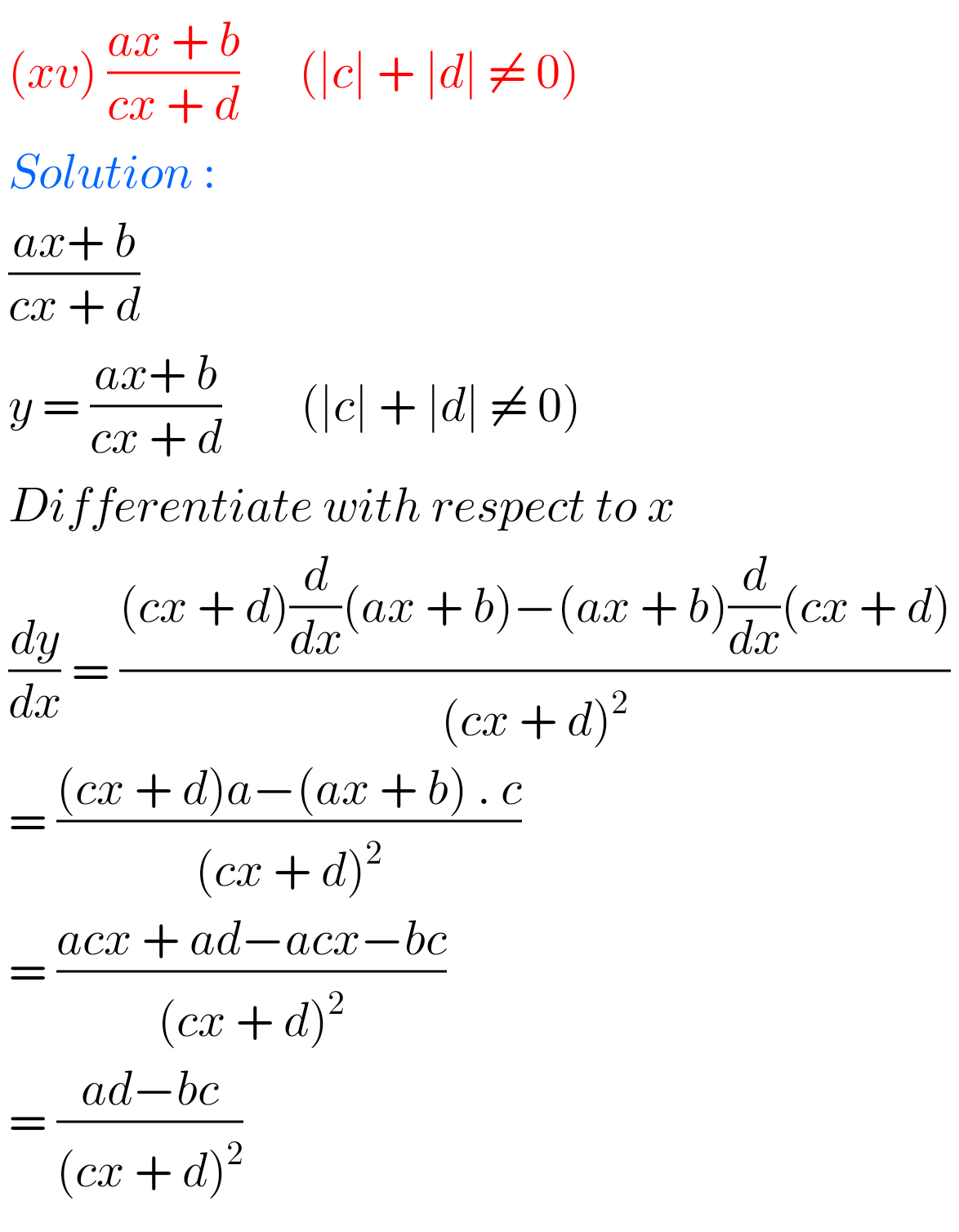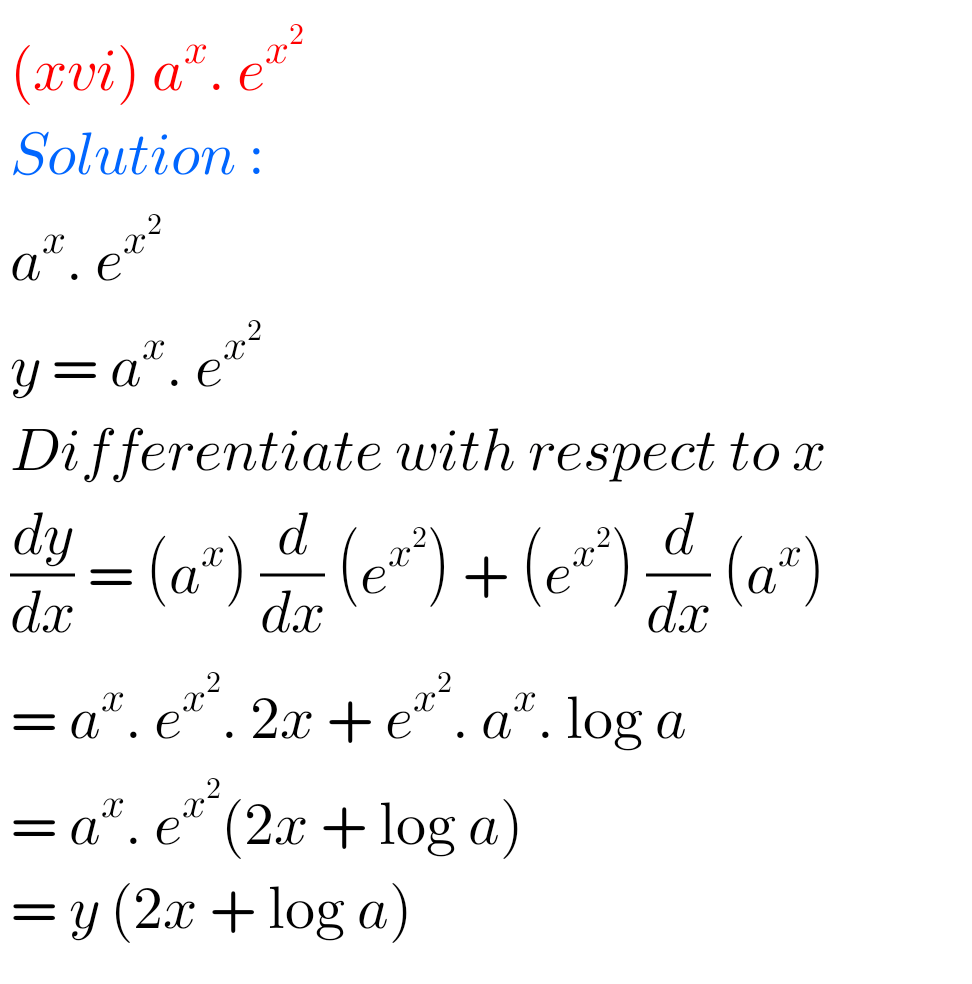## Solutions for Differentiation Inter 1st year maths 1B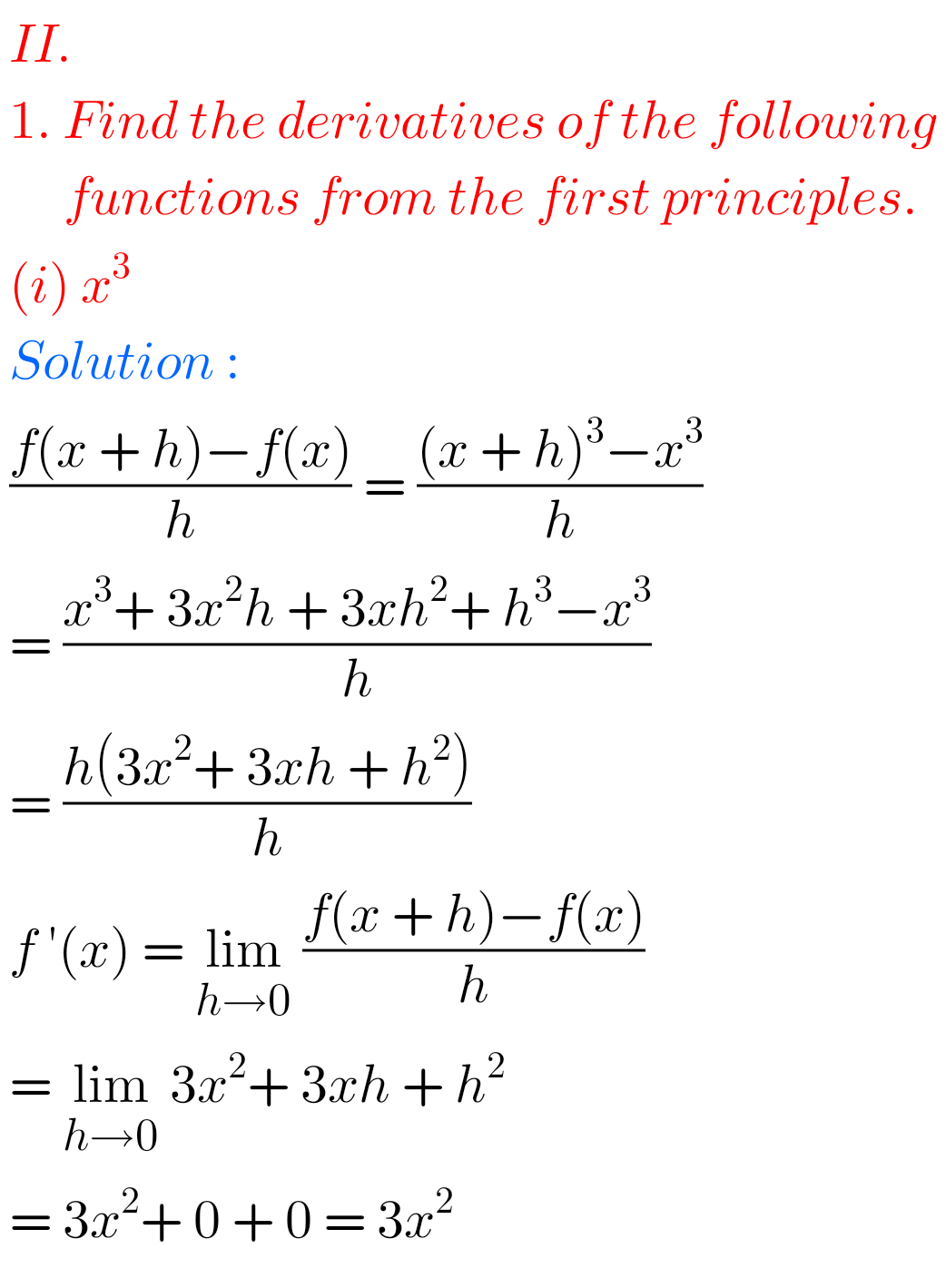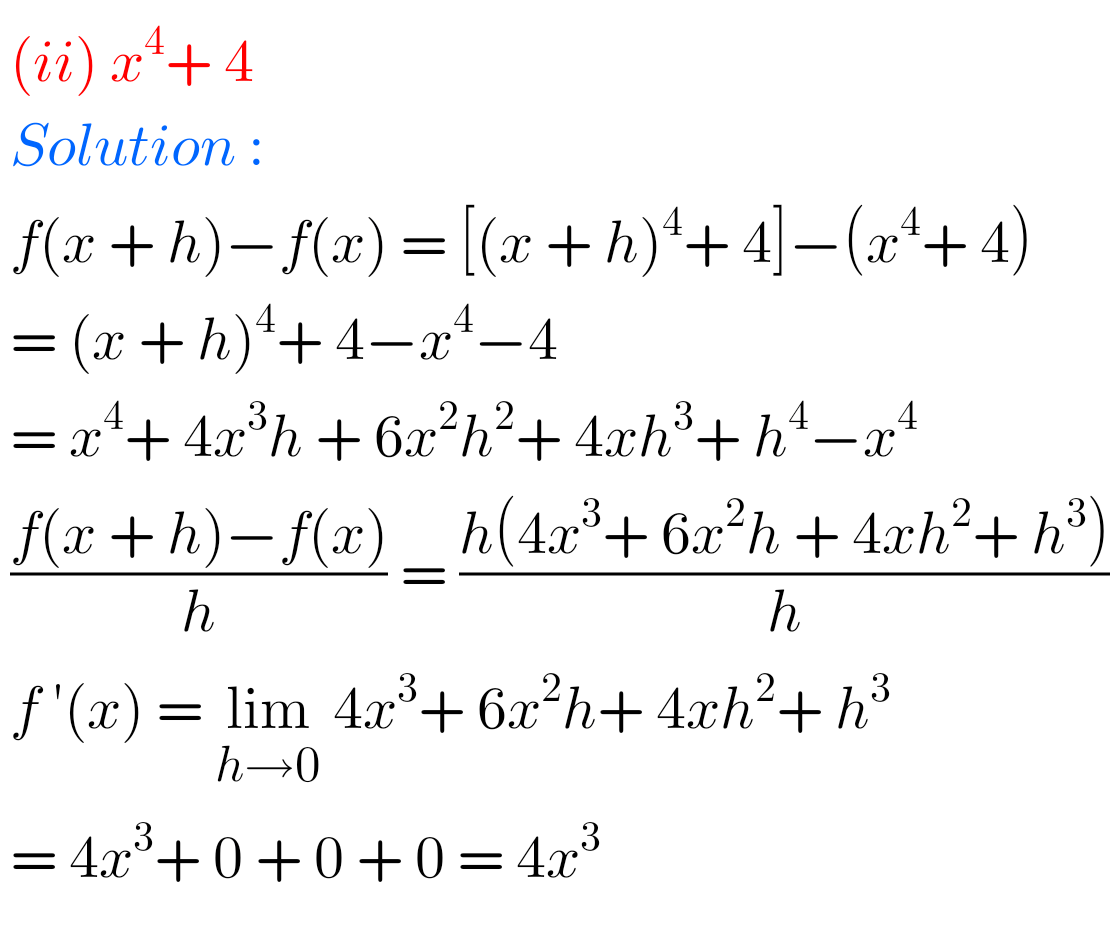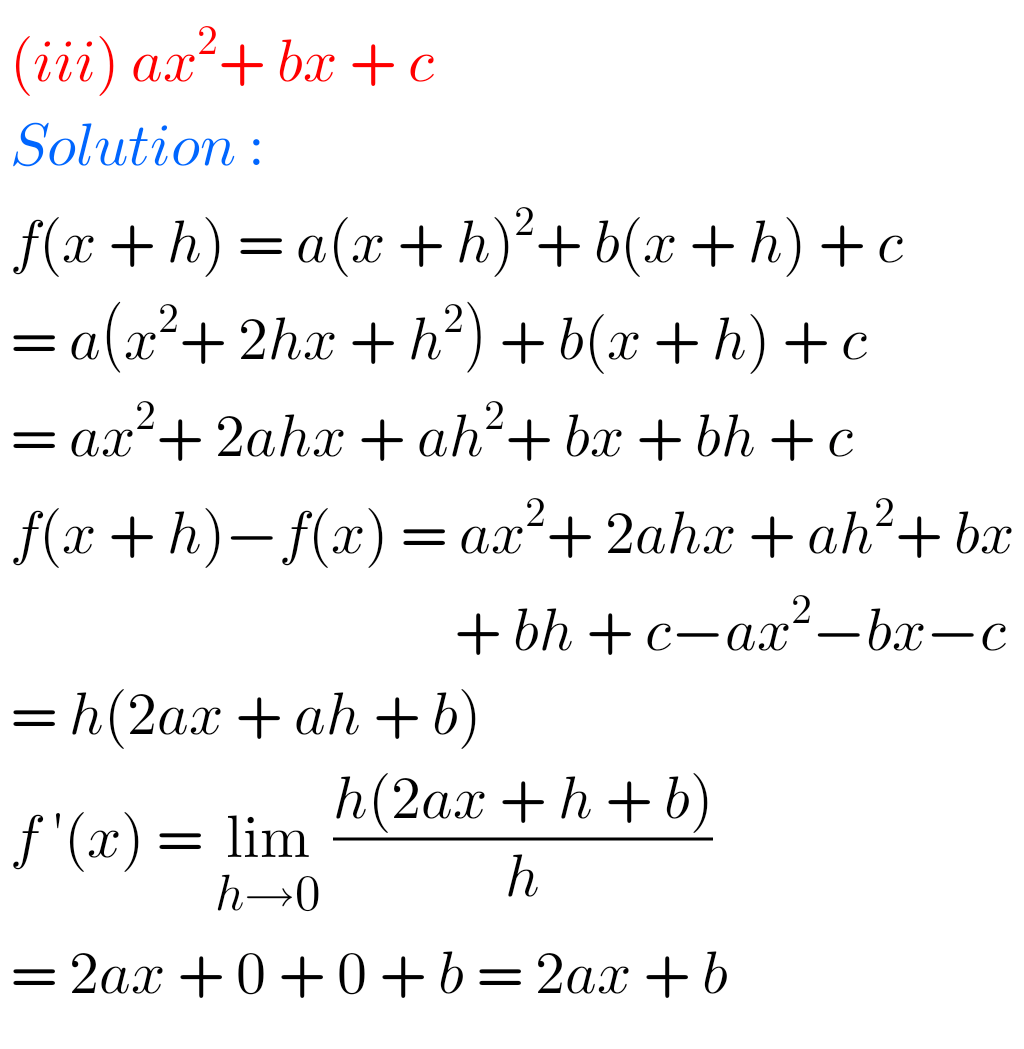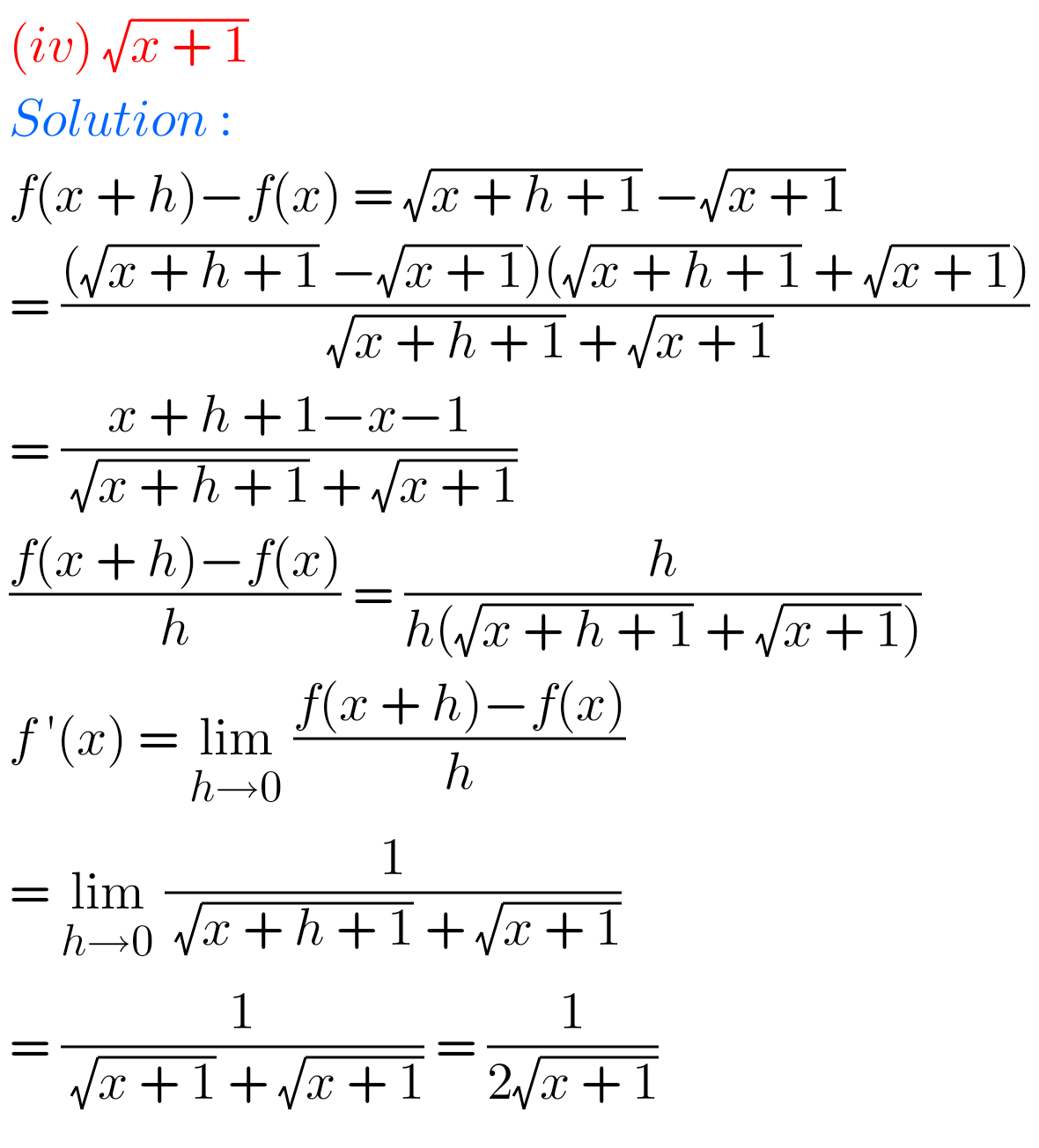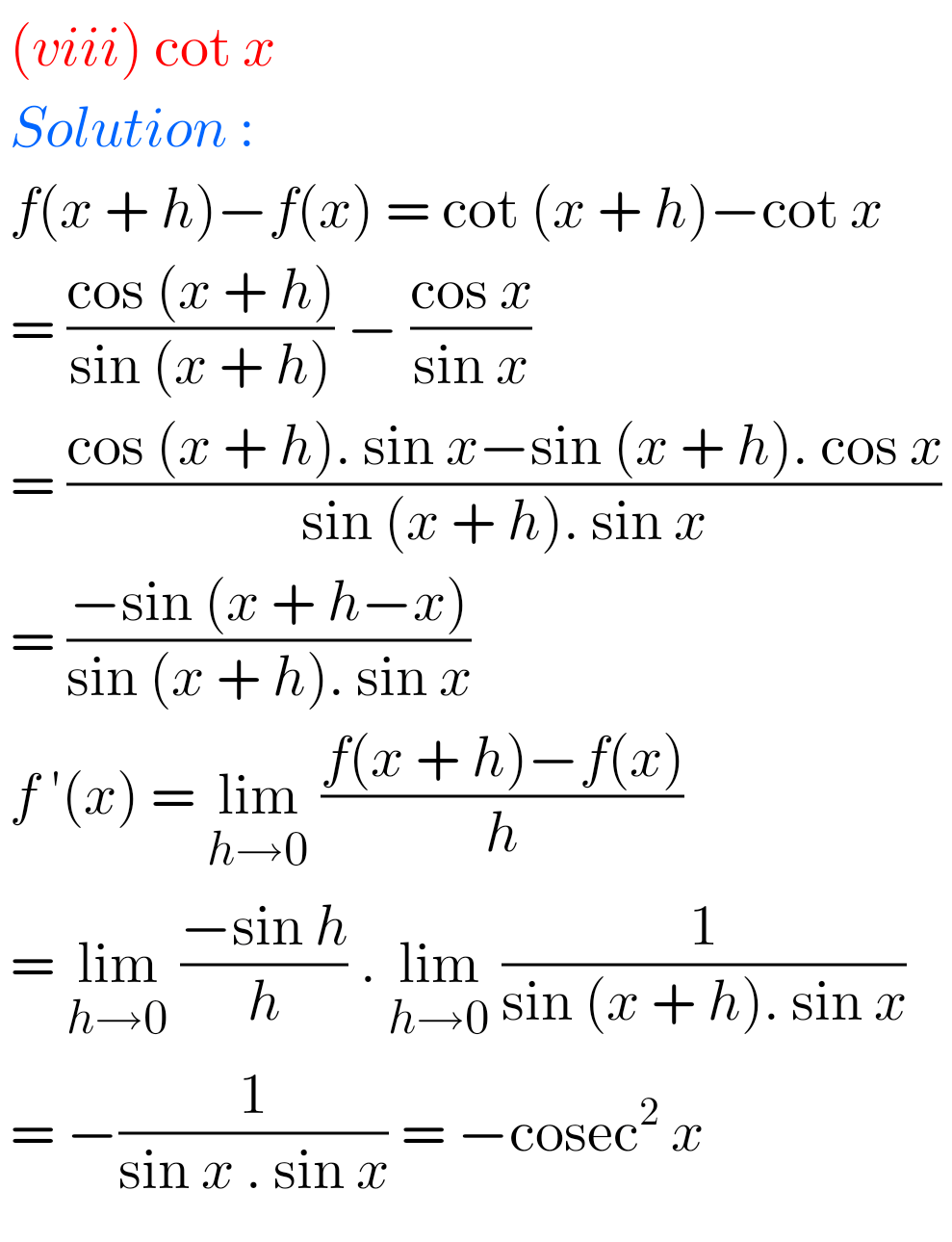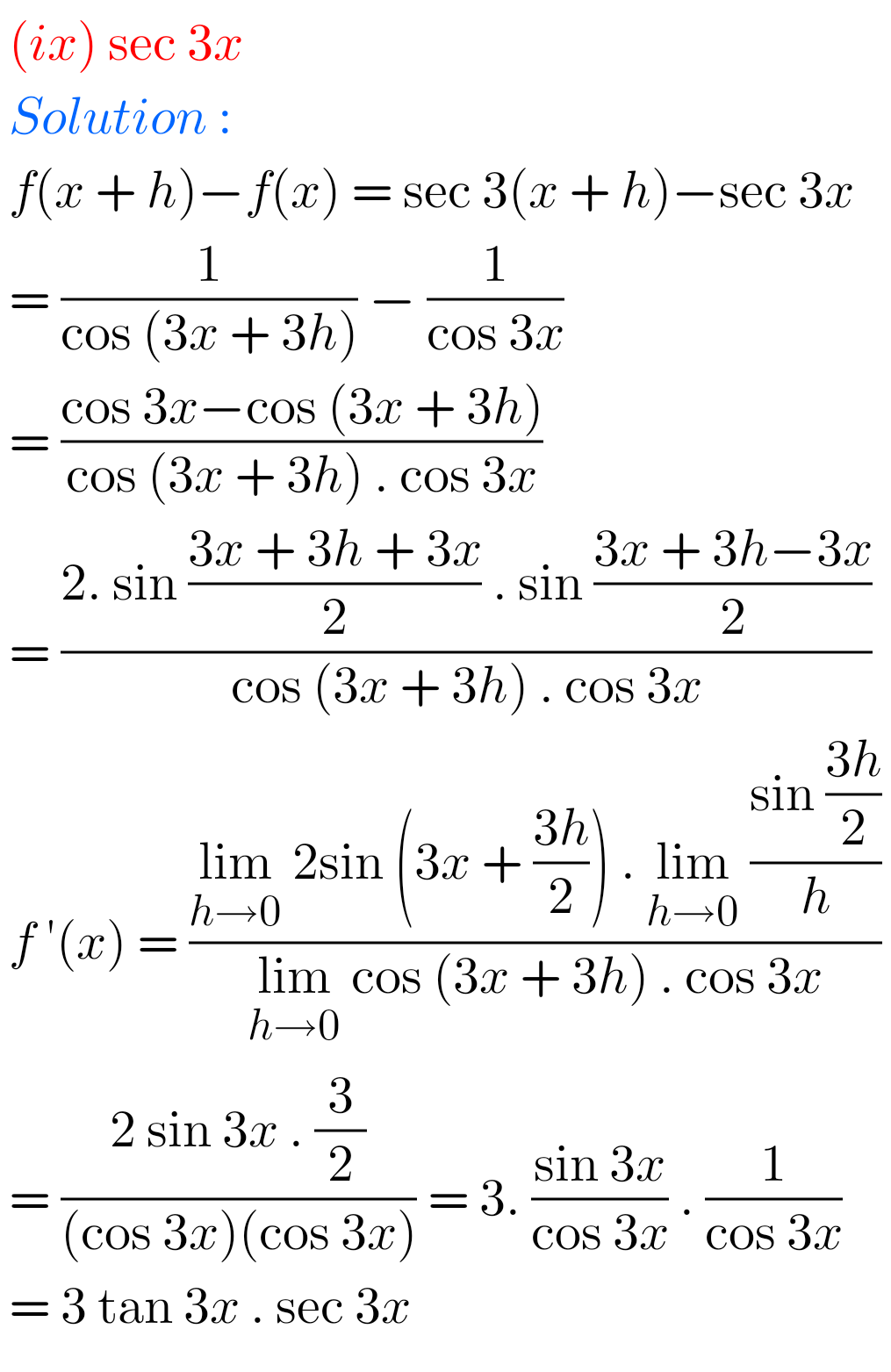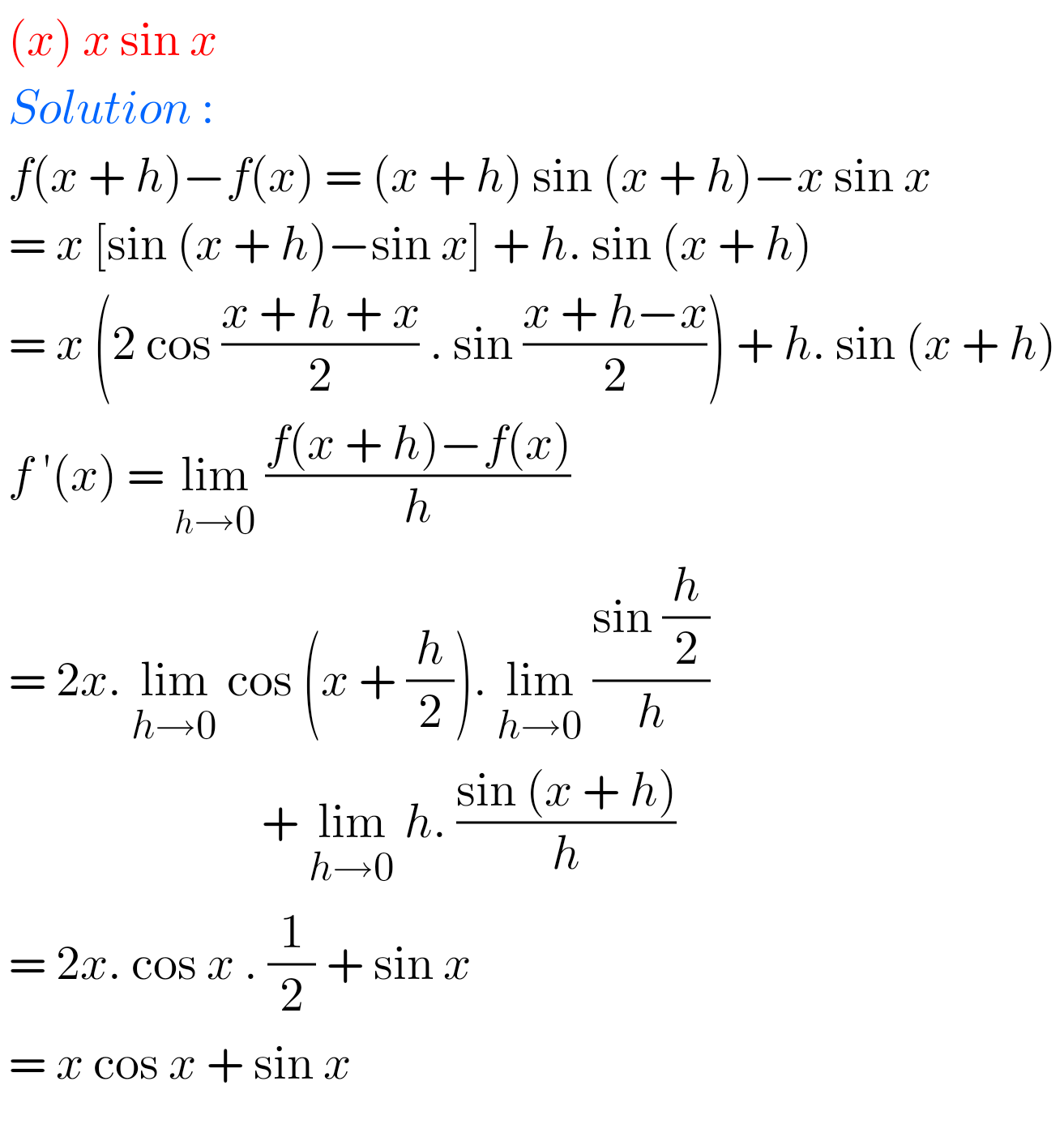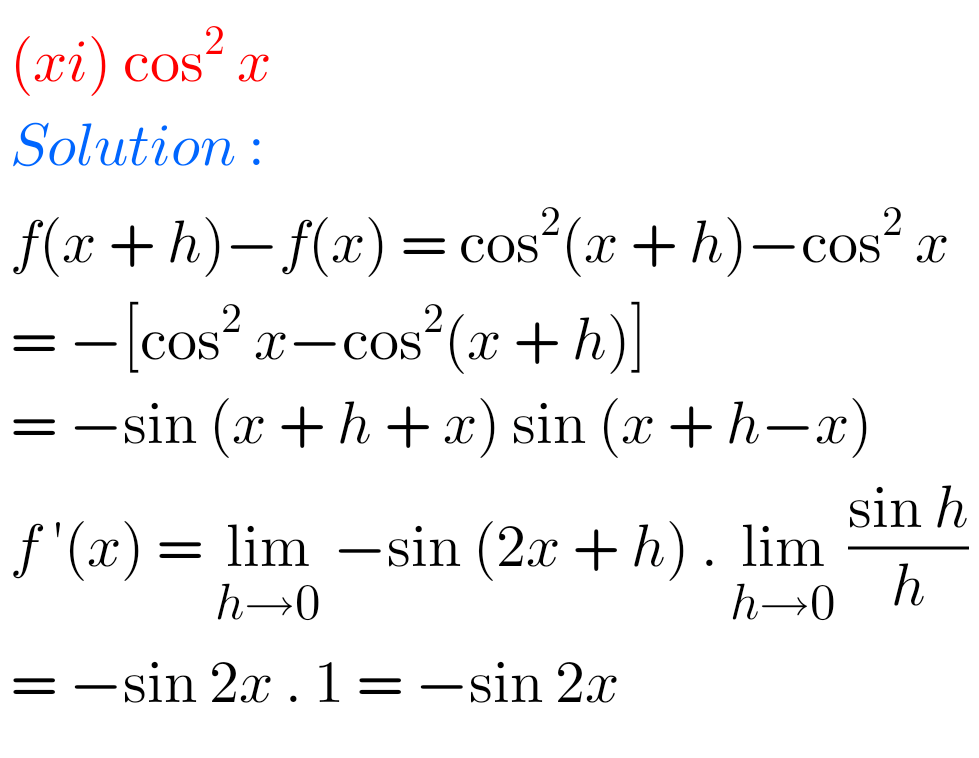## Maths solutions for Differentiation Inter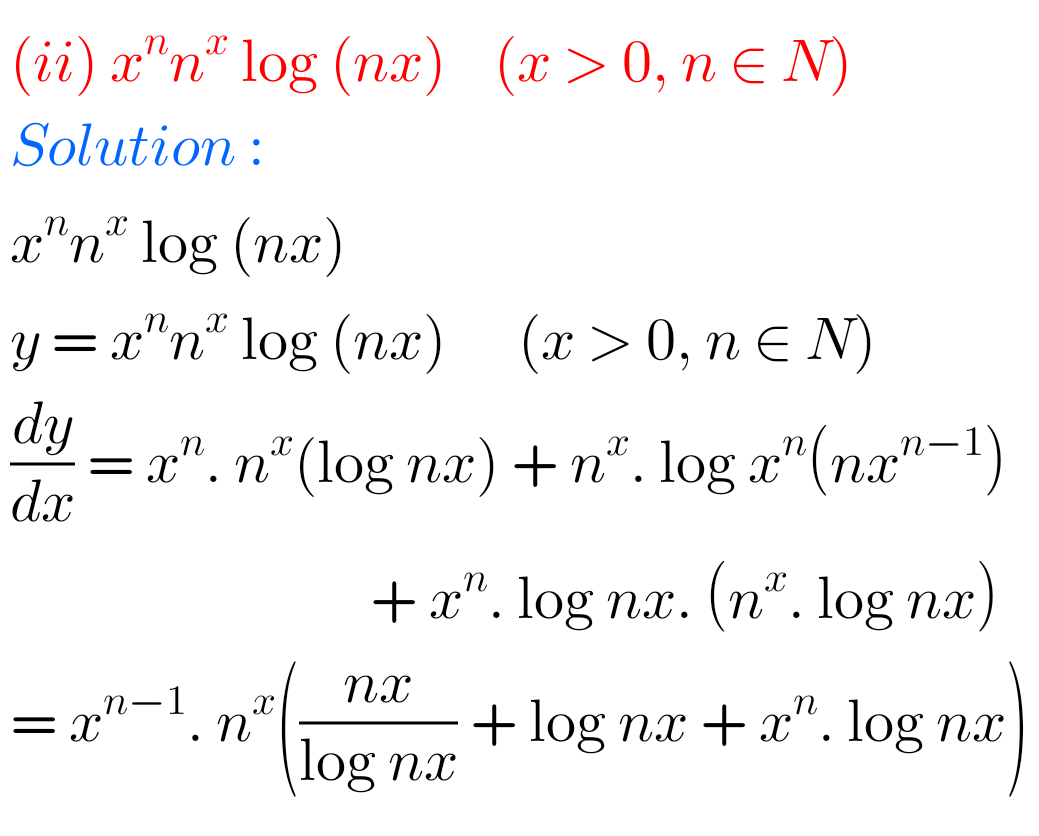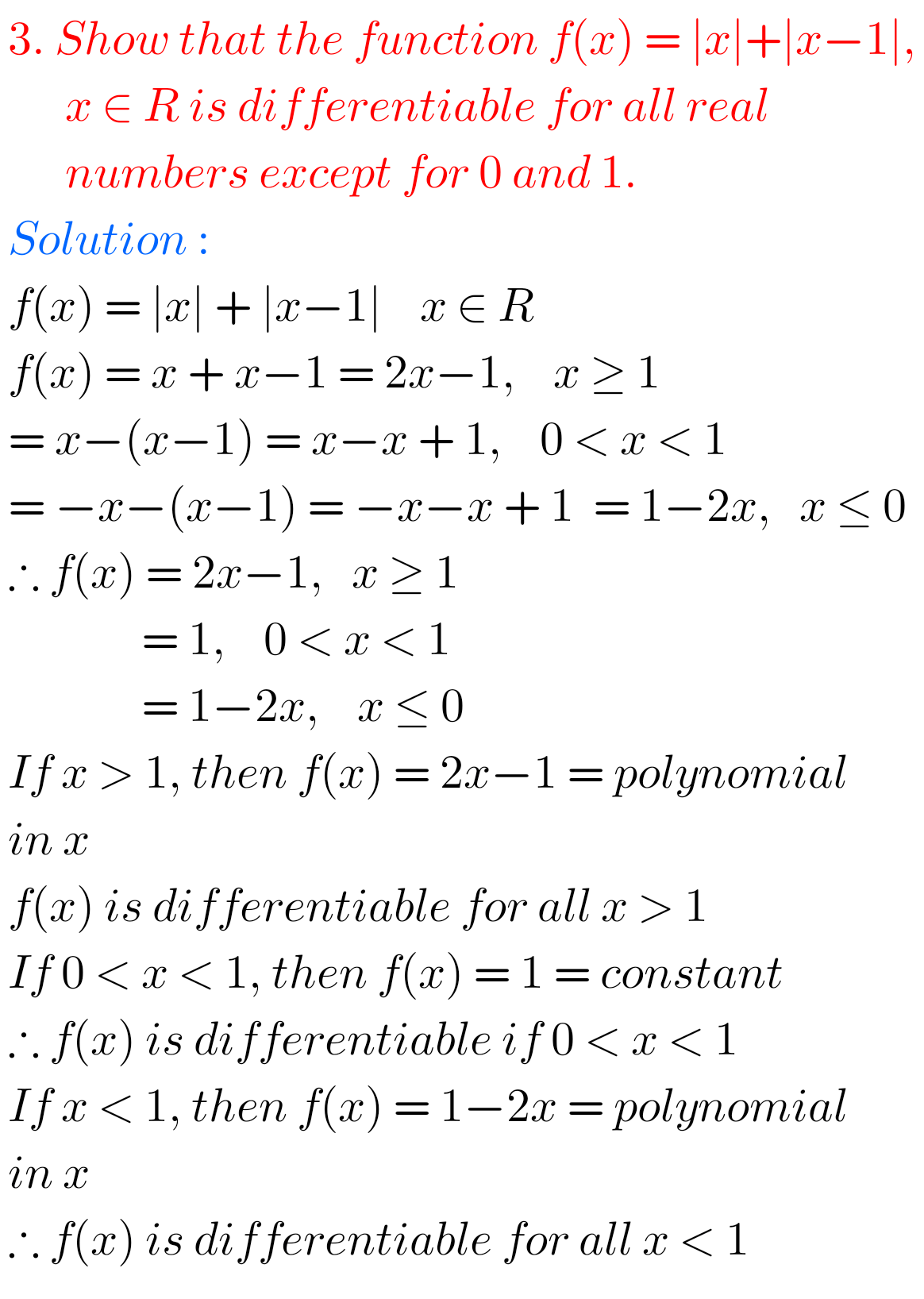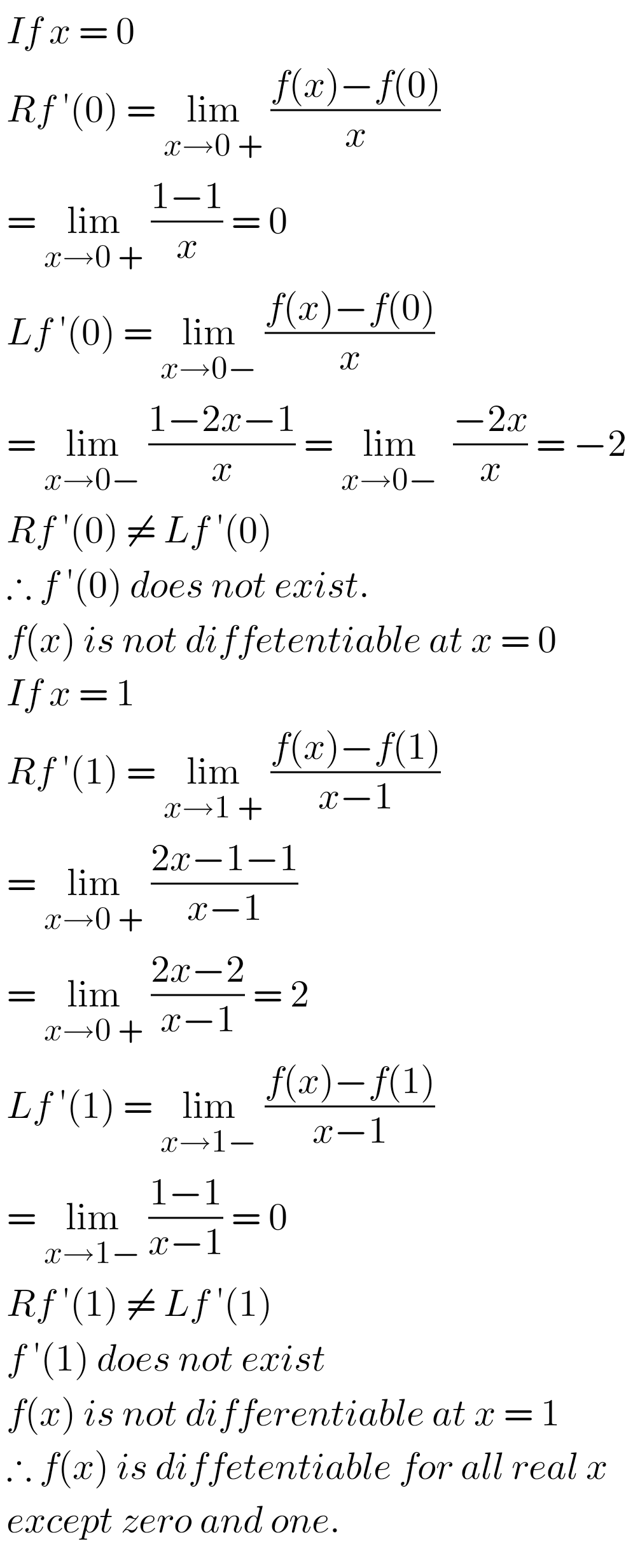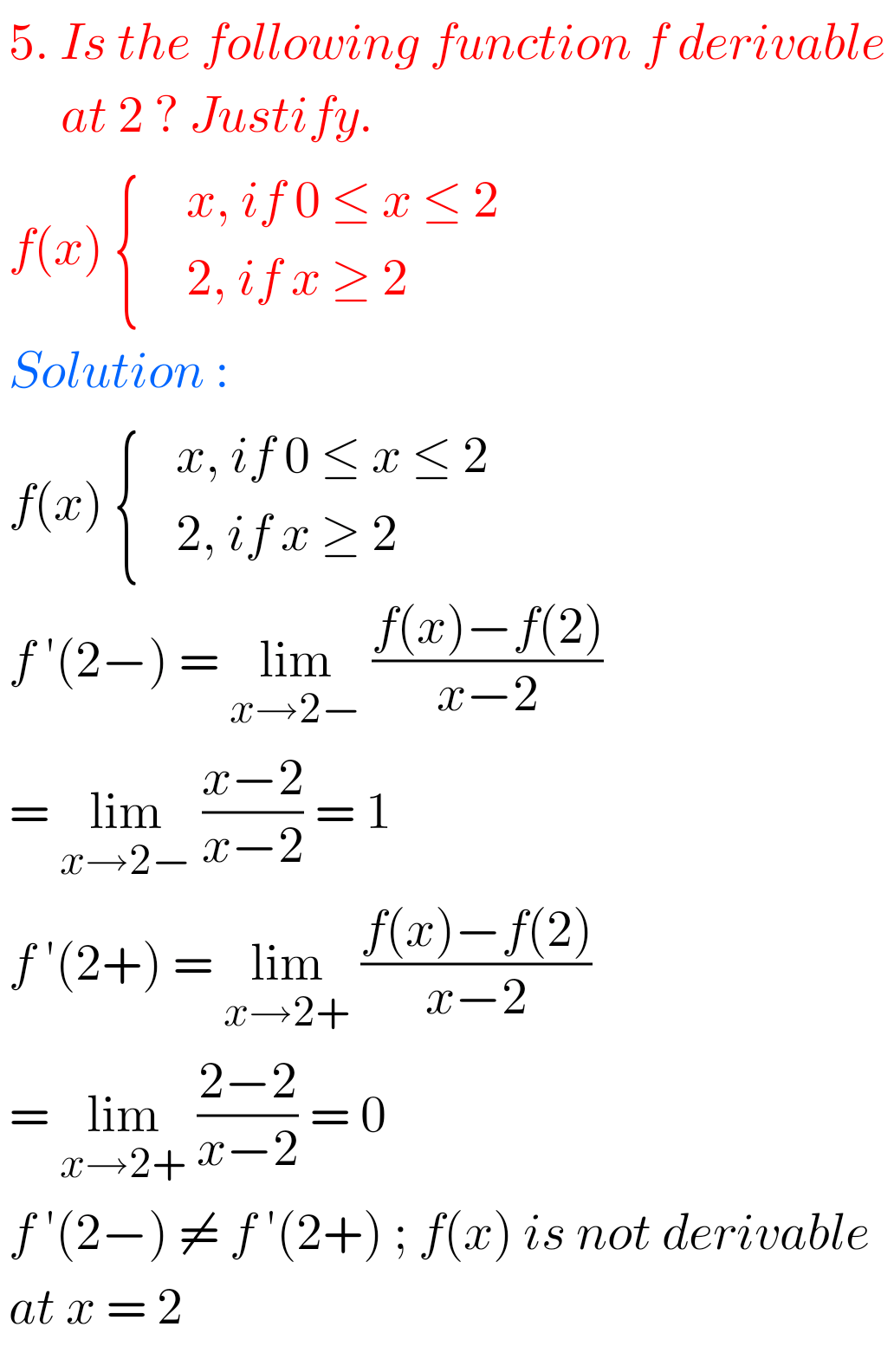Note : Observe the solutions and try them in your own method.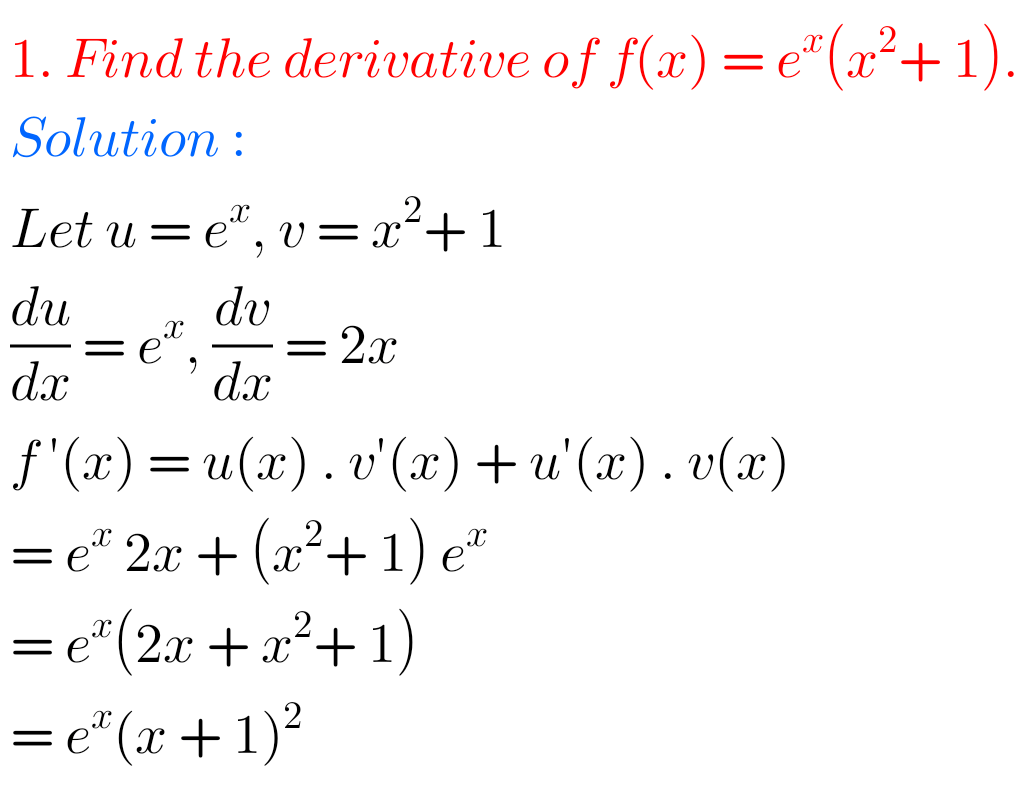SSC Maths solutions class 10